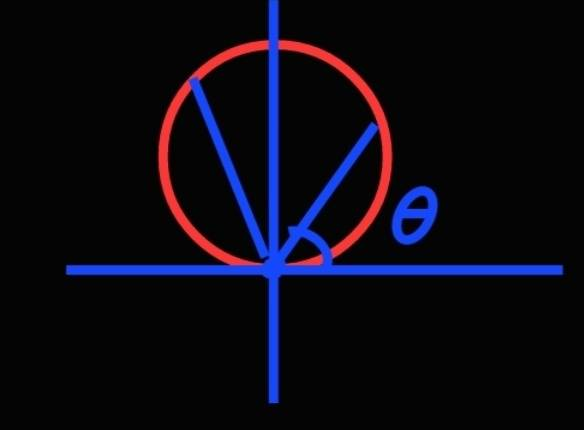# What's wrong? Electric potential of a point on a ring

• yucheng

#### yucheng

Homework Statement
N/A
Relevant Equations
N/ASo I have a ring(red) of uniform charge ##\lambda## per unit length, and I want to calculate the electric potential at the origin (actually on any point of the ring). It is clear that the ring is given by the equation $$r=2 R \sin \theta$$, in polar coordinates, where R is the radius of the ring. Since potential is given by $$V = \frac{1}{4 \pi \epsilon_0} \int \frac{dq}{r}$$, and $$dq = d\ell \lambda$$, but $$d\ell = \sqrt{(\frac{dr}{d\theta})^2 + r^2} d\theta = 2R d\theta$$. Therefore, $$V = \frac{1}{4 \pi \epsilon_0} \int \frac{d\ell \lambda}{r} = \frac{1}{4 \pi \epsilon_0} \int^{\pi}_{0} \frac{2R\lambda}{2R \sin \theta} d\theta = \frac{1}{4 \pi \epsilon_0} \lambda \int^{\pi}_{0} \frac{1}{\sin \theta} d\theta$$, which does not converge... what's wrong?

####

•Delta2

Yes because the ring is infinitesimally thin and has finite charge density ##\lambda## the electric field (and potential) is infinite for points on the ring circumference. Electric field and Potential are well defined for points on the interior or the exterior of the ring though.
Hold on for a minute while I locate a previous thread where this was discussed in more detail.

Here it is

My explanation is that we know from Gauss's law in differential form that $$\nabla\cdot\vec{E}=\frac{\rho}{\epsilon_0}$$ and $$\nabla^2 V=-\frac{\rho}{\epsilon_0}$$.

Where ##\rho## is the volume (not the linear) charge density. Because the ring is infinitesimally thin the ##\rho## of the ring become infinite for points on the ring, hence from the above two equations, electric field and potential become infinite too.

•yucheng
My explanation is that we know from Gauss's law in differential form that $$\nabla\cdot\vec{E}=\frac{\rho}{\epsilon_0}$$ and $$\nabla^2 V=-\frac{\rho}{\epsilon_0}$$.

Where ##\rho## is the volume (not the linear) charge density. Because the ring is infinitesimally thin the ##\rho## of the ring become infinite for points on the ring, hence from the above two equations, electric field and potential become infinite too.
Uh oh! I spent quite some time wondering where did I get it wrong... Luckily I asked. thanks!

•Delta2
Because the ring is infinitesimally thin the ρ of the ring become infinite for points on the ring
Oh yes, just curious, for surface charge density, like a thin sheet of charge, we also assume that it's infinitesimally thin. Does this also mean that ##\rho## is infinite?

•Delta2
My explanation is that we know from Gauss's law in differential form that $$\nabla\cdot\vec{E}=\frac{\rho}{\epsilon_0}$$ and $$\nabla^2 V=-\frac{\rho}{\epsilon_0}$$.

Where ##\rho## is the volume (not the linear) charge density. Because the ring is infinitesimally thin the ##\rho## of the ring become infinite for points on the ring, hence from the above two equations, electric field and potential become infinite too.
This argument is unfortunately invalid as it does not work for a surface charge where the volume charge density becomes formally infinite at the surface, but the potential is well defined.

Instead, we need to argue how the potential behaves as we get closer to the wire. As we approach a line charge and the distance ##\ell## to the charge is much smaller than the curvature radius of the line, the porential behaves as proportional to ##\ln(\ell)## (it must because of the divergence theorem). Therefore, the potential diverges and the field diverges as ##1/\ell##.

•Delta2
Yes ok I see now, infinity of the divergence of a field at a point doesn't necessarily imply infinity of the field at that point.

Yes ok I see now, infinity of the divergence of a field at a point doesn't necessarily imply infinity of the field at that point.
Indeed, but almost always. The exception is when the source is spread over a hypersurface of one dimension lower than the space itself. The reason is that the one-dimensional Green function of the Laplace operator does not diverge, but for any higher dimension it does

•Delta2
Indeed, but almost always
Thanks good to know that .

This argument is unfortunately invalid as it does not work for a surface charge where the volume charge density becomes formally infinite at the surface, but the potential is well defined.

Instead, we need to argue how the potential behaves as we get closer to the wire. As we approach a line charge and the distance ##\ell## to the charge is much smaller than the curvature radius of the line, the porential behaves as proportional to ##\ln(\ell)## (it must because of the divergence theorem). Therefore, the potential diverges and the field diverges as ##1/\ell##.

Indeed, but almost always. The exception is when the source is spread over a hypersurface of one dimension lower than the space itself. The reason is that the one-dimensional Green function of the Laplace operator does not diverge, but for any higher dimension it does

Are there any discussions (in books, articles) about these? The closest I found is Jackson pg. 32 the paragraph below equation (1.23) "For colume or surface distributions of charge, the potential is everywhere continuous... from the fact that E is bounded". Maybe one can carry out the limiting procedures themselves to verify, as suggested by Jackson...